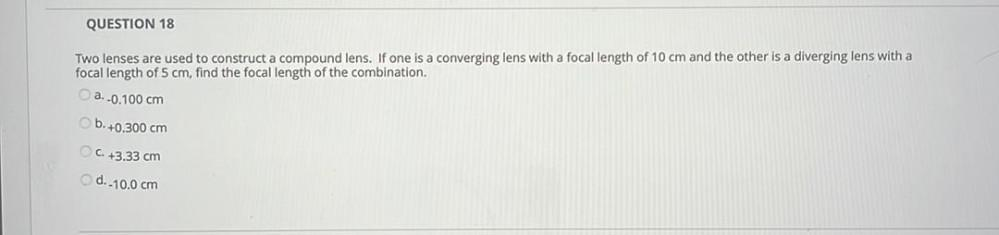Question:

# QUESTION 18 Two lenses are used to construct a compound lens. If one is a converging lens with a focal length of 10 cm and the oQUESTION 18 Two lenses are used to construct a compound lens. If one is a converging lens with a focal length of 10 cm and the other is a diverging lens with a focal length of 5 cm, find the focal length of the combination, a. -0.100 cm b. +0.300 cm C. +3.33 cm d.-10.0 cm